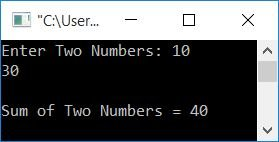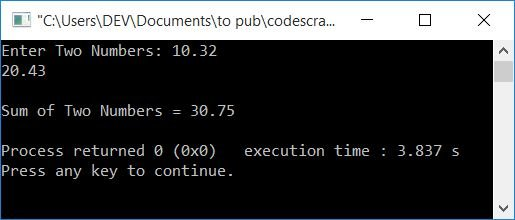# C++ Program to Add Two Numbers Using a Pointer

To add two numbers using a pointer in C++ programming, you have to ask the user to enter the two numbers. Then make two pointer-type variables of the same type, say *ptr1 and *ptr2, to initialize the addresses of both variables (that hold numbers), and using another variable, say sum, store the addition of the two numbers, i.e., sum = *ptr1 + *ptr2, and display the value of sum on the screen.

Here, * denotes a value at the operator, and & denotes the operator's address.

The following C++ program prompts the user to enter two numbers in order to sum them using a pointer. And then display the result of the addition on the screen:

```#include<iostream>
using namespace std;
int main()
{
int num1, num2, *ptr1, *ptr2, sum=0;
cout<<"Enter Two Numbers: ";
cin>>num1>>num2;
ptr1 = &num1;
ptr2 = &num2;
sum = *ptr1 + *ptr2;
cout<<"\nSum of Two Numbers = "<<sum;
cout<<endl;
return 0;
}```

This program was built and runs under the Code::Blocks IDE. Here is its sample run:Now supply any two numbers, say 10 and 30, as input. Press the ENTER key to see the addition of two given numbers using the pointer as shown in the final snapshot of the sample run given below:Here, the address of the first number (stored in num1) gets initialized to ptr1, whereas the address of the second number gets initialized to ptr2 using the & (address of) operator.

And using the * (value at address) operator, we've added the two numbers and initialized it to sum. Finally, just print the value of it as output.

### What if the number has a decimal point?

To handle a real number, just change the data type from int to float. That is, the following statement:

`int num1, num2, *ptr1, *ptr2, sum=0;`

gets replaced with:

`float num1, num2, *ptr1, *ptr2, sum=0;`

Rest of the things will be the same. And here is its sample run on real-number input:#### The same program in different languages

C++ Quiz

« Previous Program Next Program »